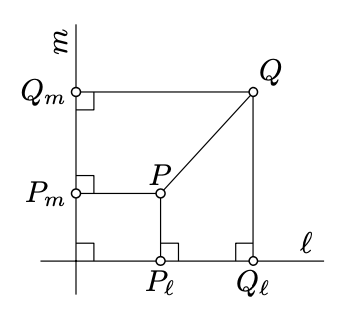# 7.6: Method of coordinates

$$\newcommand{\vecs}{\overset { \rightharpoonup} {\mathbf{#1}} }$$ $$\newcommand{\vecd}{\overset{-\!-\!\rightharpoonup}{\vphantom{a}\smash {#1}}}$$$$\newcommand{\id}{\mathrm{id}}$$ $$\newcommand{\Span}{\mathrm{span}}$$ $$\newcommand{\kernel}{\mathrm{null}\,}$$ $$\newcommand{\range}{\mathrm{range}\,}$$ $$\newcommand{\RealPart}{\mathrm{Re}}$$ $$\newcommand{\ImaginaryPart}{\mathrm{Im}}$$ $$\newcommand{\Argument}{\mathrm{Arg}}$$ $$\newcommand{\norm}{\| #1 \|}$$ $$\newcommand{\inner}{\langle #1, #2 \rangle}$$ $$\newcommand{\Span}{\mathrm{span}}$$ $$\newcommand{\id}{\mathrm{id}}$$ $$\newcommand{\Span}{\mathrm{span}}$$ $$\newcommand{\kernel}{\mathrm{null}\,}$$ $$\newcommand{\range}{\mathrm{range}\,}$$ $$\newcommand{\RealPart}{\mathrm{Re}}$$ $$\newcommand{\ImaginaryPart}{\mathrm{Im}}$$ $$\newcommand{\Argument}{\mathrm{Arg}}$$ $$\newcommand{\norm}{\| #1 \|}$$ $$\newcommand{\inner}{\langle #1, #2 \rangle}$$ $$\newcommand{\Span}{\mathrm{span}}$$$$\newcommand{\AA}{\unicode[.8,0]{x212B}}$$

The following exercise is important; it shows that our axiomatic definition agrees with the model definition.

## Exercise $$\PageIndex{1}$$Let $$\ell$$ and $$m$$ be perpendicular lines in the Euclidean plane. Given a point $$P$$, let $$P_{\ell}$$ and $$P_m$$ denote the foot points of $$P$$ on $$\ell$$ and $$m$$ respectively.

1. Show that for any $$X \in \ell$$ and $$Y \in m$$ there is a unique point $$P$$ such that $$P_{\ell} = X$$ and $$P_m = Y$$.
2. Show that $$PQ^2 = P_{\ell}Q_{\ell}^2 + P_m Q_m^2$$ for any pair of points $$P$$ and $$Q$$.
3. Conclude that the plane is isometric to $$(\mathbb{R}^2, d_2)$$.
Hint

(a). Use the uniqueness of the parallel line (Theorem 7.1.1).

(b). Use Lemma 7.5.1 and the Pythagorean theorem (Theorem 6.2.1)

Once this exercise is solved, we can apply the method of coordinates to solve any problem in Euclidean plane geometry. This method is powerful and universal; it will be developed further in Chapter 18.

## Exercise $$\PageIndex{2}$$

Use the Exercise $$\PageIndex{1}$$ to give an alternative proof of Theorem 3.5.1 in the Euclidean plane.

That is, prove that given the real numbers $$a, b$$, and $$c$$ such that

$$0 < a \le b \le c \le a + b$$,

there is a triangle $$ABC$$ such that $$a = BC$$, $$b = CA$$, and $$c = AB$$.

Hint

Set $$A = (0, 0), B = (c, 0)$$, and $$C = (x, y)$$. Clearly, $$AB = c$$, $$AC^2 = x^2 + y^2$$ and $$BC^2 = (c - x)^2 + y^2$$.

It remains to show that there is a pair of real numbers $$(x, y)$$ that satisfy the following system of equations:

$$\begin{cases} b^2 = x^2 + y^2 \\ a^2 = (c- x)^2 + y^2 \end{cases}$$

if $$0 < a \le b \le c \le a + c$$.

## Exercise $$\PageIndex{3}$$

Consider two distinct points $$A = (x_A, y_A)$$ and $$B = (x_B, y_B)$$ on the coordinate plane. Show that the perpendicular bisector to $$[AB]$$ is described by the equation

$$2 \cdot (x_B - x_A) \cdot x + 2 \cdot (y_B - y_A) \cdot y = x_B^2 + y_B^2 - x_A^2 - y_B^2$$.

Conclude that line can be defined as a subset of the coordinate plane of the following type:

1. Solutions of an equation $$a \cdot x + b \cdot y = c$$ for some constants $$a, b$$, and $$c$$ such that $$a \ne 0$$ or $$b \ne 0$$.
2. The set of points $$(a \cdot t + c, b \cdot t + d)$$ for some constants $$a, b, c$$, and $$d$$ such that $$a \ne 0$$ or $$b \ne 0$$ and all $$t \in \mathbb{R}$$.
Hint

Note that $$MA = MB$$ if and only if

$$(x - x_A)^2 + (y - y_A)^2 = (x - x_B)^2 + (y - y_B)^2$$

where $$M = (x, y)$$. To prove the first part, simplify this equation. For the remaining parts use that any line is a perpendicular bisector to some line segment.

This page titled 7.6: Method of coordinates is shared under a CC BY-SA 4.0 license and was authored, remixed, and/or curated by Anton Petrunin via source content that was edited to the style and standards of the LibreTexts platform; a detailed edit history is available upon request.